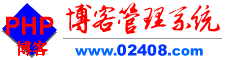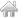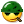免费个人博客管理系统

发表新话题免费个人博客管理系统网络技术免费PHP教程正文

PHP连接MySQL数据库的5种方法

<?php
header("Content-Type:text/html; charset=utf-8"); // 设置文档编码，防止出现乱码情况，与MySQL数据库连接没有直接关系
\$servername = "localhost"; //改成自己的mysql数据库服务器
\$username = "root"; //改成自己的mysql数据库用户名
\$password = "123456"; //改成自己的mysql数据库密码
\$dbname = "test"; //改成自己的mysql数据库名
\$select_db = mysql_select_db(\$dbname); // 连接到指定数据库
if (!\$select_db) {
die("<h2>无法连接到数据库:</h2>" . mysql_error());
}
mysql_query("SET NAMES utf8"); // 设置数据库编码
//数据库查询代码操作开始
\$sql = "select * from db_table";
\$res = mysql_query(\$sql);
if (!\$res) {
die("could get the res:n" . mysql_error());
}
while (\$row = mysql_fetch_assoc(\$res)) {
print_r(\$row);
}
//查询结束
//在所有增删改查结束后，关闭数据库连接
mysql_close(\$con);

<?php
\$servername = 'localhost'; //改成自己的mysql数据库服务器
\$username = 'root'; //改成自己的mysql数据库用户名
\$dbname = 'test'; //改成自己的mysql数据库名
//连接数据库错误提示
if (mysqli_connect_errno(\$conn)) {
die("连接 MySQL 失败: " . mysqli_connect_error());
}
mysqli_query(\$conn,"set names utf8"); //数据库编码格式
//数据库查询代码操作开始
\$sql = “select * from db_table”;
\$query = mysqli_query(\$conn,\$sql);
while(\$row = mysqli_fetch_array(\$query)){
echo \$row['title'];
}
//查询结束
//在所有增删改查结束后，关闭MySQL数据库连接
mysqli_close(\$conn);

<?php
//连接数据库方式1
\$conn = new mysqli('localhost', 'root', 'password', 'test');

//连接数据库方式2
// \$conn = new mysqli();
// \$conn -> connect('localhost', 'root', 'password', 'test');

//check connection (检查PHP是否连接上MYSQL)
if (\$conn -> connect_errno) {
printf("Connect failed: %sn", \$conn->connect_error);
exit();
}
//数据库查询代码操作开始
\$sql = “select * from db_table”;
\$query = \$conn->query(\$sql);
while(\$row = \$query->fetch_array()){
echo \$row['title'];
}
//释放结果集
\$query1 -> free_result();
//在所有增删改查结束后，关闭MySQL连接
\$conn -> close();

<?php
\$db = new PDO('mysql:host=localhost;dbname=test', 'root', 'password');
try {
foreach (\$db->query('select * from db_table') as \$row){
print_r(\$row);
}
\$db = null; //关闭数据库
} catch (PDOException \$e) {
echo \$e->getMessage();
}
?>

<?php
//安装驱动-引入类即可
\$conn->Execute("set names utf8");
}
?>
//关闭连接
\$conn->close();

最新回答

评论专区：添加表情

更多 >